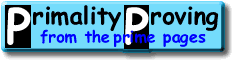Finding primes & proving primality 4.1 Neoclassical tests: APR and APR-CLHome > Primality Proving > Chapter Four > APR and APR-CL

How do we improve on the tests of the previous chapter?  The way Williams and others began in the 70's was to use the factors of other polynomials of n such as n2+1, n2+n+1 and n2-n+1 [Williams78].  But why stop there?  Why not try nm-1 for a higher exponent m such as 5040, then every prime q such that q-1 divides 5040 (which does not divide n) must divide n5040-1 (by Fermats Little Theorem).

This (with much more cleverness) makes (for m = 5040) a product of primes q which is greater than 1052.  So after we show there are theorems similar to the classical theorems which only require a factorization to the square root of n, then using this same m, 5040, we will be done for all numbers with less than 100 digits--without any (explicit) factoring (the same q's work for all of these n's).

What about even larger N's?  It is always possible to find the necessary factors.  In fact it has been shown that there is always an integer m with

m < (log n)log log log n
for which the factors q dividing nm-1 with q-1 dividing m, have a product at least the size of the square root of n. Usually m is around 100,000,000 for numbers n with about 3,000 digits.

This is roughly (very roughly!) how Adleman, Pomerance and Rumely began the modern age of primality testing by introducing the APR primality test [APR83] in 1979.  The running time of their method is almost polynomial--its running time t is bounded as follows

(log n)(c1 log log log n) < t < (log n)(c2 log log log n)

(recognize those bounds?)

Soon Cohen and Lenstra [CL84] improved this test into a practical version called APRT-CL that handles 100 digit numbers in a matter of seconds (see also [CL87], [Mihailescu98], and [BH90]).  (They improved it by replacing the general reciprocity law for the power residue symbol with much easier to calculate Jacobi sums.)

It is also possible to mix the two approaches (the classical using large factors of n±1 and the neoclassical above using many small factors of nm-1).  One example of this mixed approach is Tony Forbes VFYPR (limited to 2982 digits).

 [ next page | previous page ] Copyright © 2023 PrimePages (Originally written by Chris Caldwell)